#5

## 寫程式實作Eratosthenes 篩法建質數表時，發現了質數在自然數中的密度趨近於0的證明

1. LeetCode- 204. Count Primes- 計算小於n的質數有幾個
2. CodeWar- Prime Streaming (PG-13)- 由小至大快速產生質數

``````import math

#find all primes less than n
def findPrime(n):
if n<=2:
return []
isPrime = [True]*n
for i in range(3,int(math.sqrt(n))+1,2):
if isPrime[i]:
isPrime[i * i :: i] = [False]*len(isPrime[i * i :: i])
return +[i for i in range(3,n,2) if isPrime[i]]
``````

# 質數占自然數多少比例思考

1/2 * 2/3 * 4/5 * 6/7 * …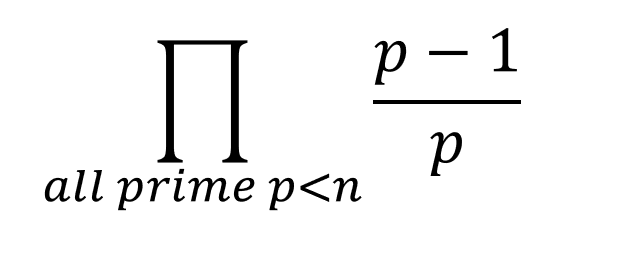## 動機: 從質數思考到宇宙

(可能因此人類一直找不到外星生物)

## 數學式證明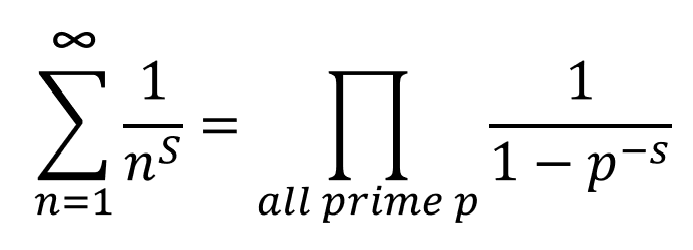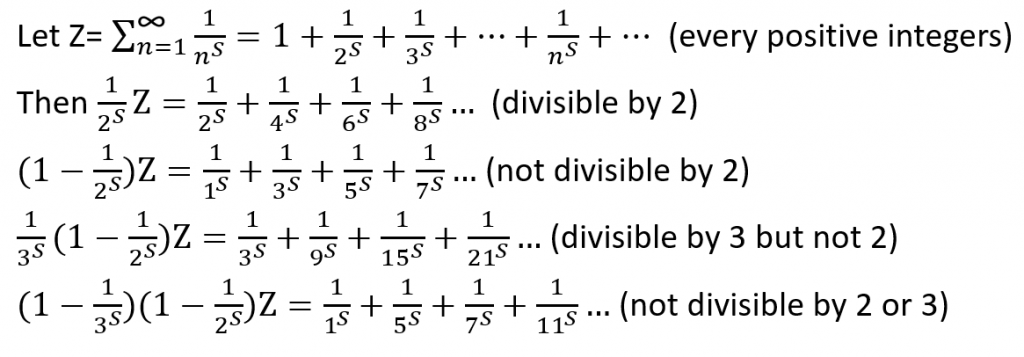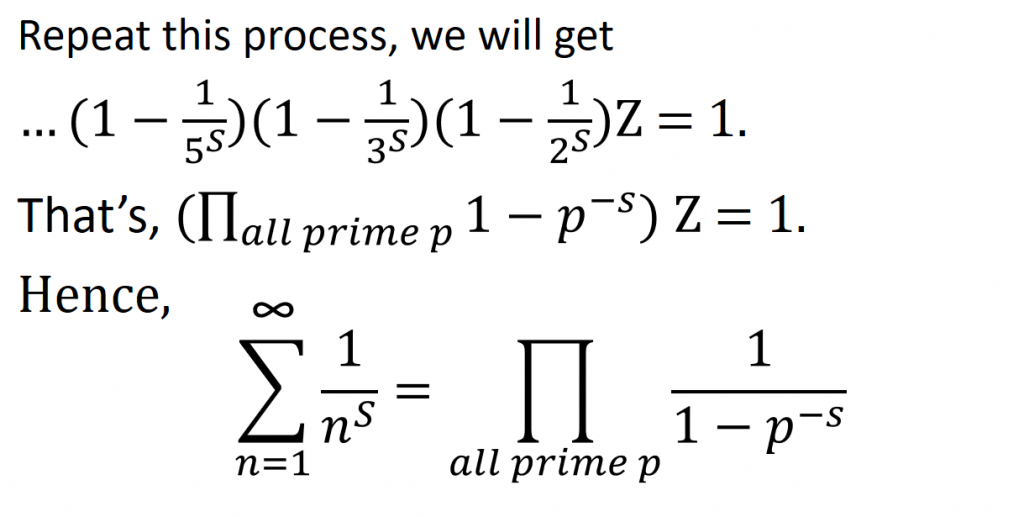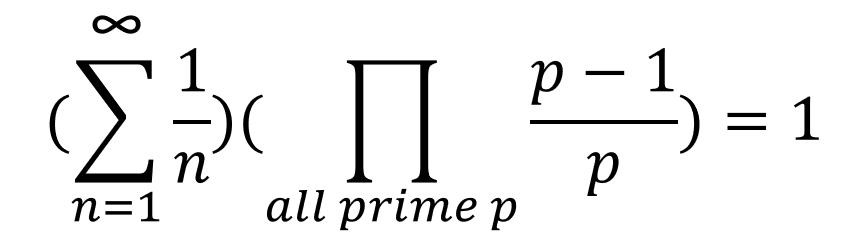``````1+1/2+1/3+…+1/n 大約就是 ln n
``````

(補充: 事後在wiki- 質數定理看到小於n的質數的比例真的大約是(1/ln n)，沒想到寫程式恰巧想到了這個結論)